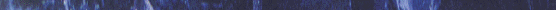Papers

The papers and their abstracts, which represent the so far published work of the author, are given below. If you have any question, please, contact the author.Journals

ConferencesAbstracts

Quaternary voltage-mode CMOS circuits for  multiple-valued logic

A set of novel voltage-mode CMOS circuits for the implementation of Multiple-Valued Logic (MVL) systems is introduced. The circuit level implementation of the Multiple-Valued Logic operators: logical sum, logical product, level-up, level-down, and level conversions are presented. The mathematical properties of the latter operator are formally proved. The proposed multiple-valued logic circuits exhibits zero static power consumption, do not use clocking, and function on any arithmetic base. The proposed circuits consist of appropriately constructed enhancement-mode and depletion-mode MOSFETs of 1.5 um technology. Simulation of the introduced quaternary logic voltage-mode CMOS circuits, using SPICE, indicates improved performance (higher speeds) compared to existing ones.Design of novel multiple-valued logic voltage-mode storage circuits

Novel multiple-valued logic (MVL) voltage-mode storage circuits, namely the dynamic and pseudo-static latch, and the dynamic and pseudo-static master-slave, using enhancement- or both enhancement- and depletion-mode MOSFETs, are proposed. These storage circuits consist of some new building units. Low power dissipation, zero static power consumption during the steady state operation and high speed characterize the proposed circuits. Additionally, we extend the use of fundamental principles of the True Single-Phase Clocked Logic approach of binary logic to MVL, which implies smaller switching activity. Considering quaternary logic, the proposed circuits are simulated by SPICE tool. The obtained result proclaims substantial improvements, in terms of power, speed, and transistor count. Adopting the principal design concepts of the proposed quaternary circuits, we developed a generalized formal methodology for systematic design of storage circuits of any radix. Both the methodology and the proposed circuits exhibit regular, modular, iterative and hierarchical designed architectures, which eventually imply regular physical implementations suitable for VLSI implementations.Quaternary voltage-mode multiple-valued logic adder circuits

Abstract is not yet available.A unified environment for digital systems simulation

Here is presented a Unified Environment for the simulation of digital systems. This Environment was designed originally for a Macintosh computer. Its main features are (a) the introduction of a third (unknown) logical state, (b) a fast simulation algorithm, (c) ability to run on small computers and (d) is designed on the principles of Open Systems.Multiple-valued logic voltage-mode storage circuits based on true-single-phase clocked logic

A number of novel voltage-mode multiple-valued logic circuits are introduced. Adopting the main features of the true single-phase clocked logic, efficient quaternary logic dynamic and pseudo-static latches, dynamic and static master-slave storage units, and uni-signal controlled pass gates are proposed. These circuits use two kinds of MOS transistors, i.e., enhancement and depletion mode, each of which has two threshold voltages. The proposed circuits exhibit regular, modular, and iterative structure, which means that the MVL circuits are VLSI implementable and can be easily re-designed for any radix of an arithmetic system. Since we use only clock signal, the derived circuits have low power dissipation. Comparisons with existing circuits prove substantial improvements in terms of speed, power consumption, and transistor count.Design methodology of multiple-valued logic voltage-mode storage circuits

A novel methodology designing for Multiple-Valued Logic voltage-mode storage circuits is introduced. Using the proposed inverter-based unit, uni-signal controlled pass gates and True Single-Phase Clocked Logic-based output units, efficient r-ary (where r is the radix) dynamic and pseudo-static latches can be designed. They exhibit regular, modular, and iterative structure, which means that the for Multiple-Valued Logic circuits are VLSI implementable. Also,  these circuits use two kinds of MOS transistors, i.e., enhancement and depletion mode. Since we use only clock signal, additional contribution to low power dissipation of the derived circuits is been made. Comparisons with existing circuits prove substantial improvements in terms of speed, power consumption, and transistor count.The design of low power multiple-valued logic encoder and decoder circuits

Novel multiple-valued logic (MVL) voltage-mode circuits, namely encoder and decoder circuits, using both enhancement- and depletion-mode MOSFETs, are introduced. High performance and low power dissipation, due to zero-static power consumption during their steady-state operation, characterize the proposed circuits. More specifically, considering quaternary logic, and 0.7 um technology, the encoder and decoder circuits are implemented and simulated by the SPICE tool. The results obtained show substantial improvements, in terms of power, speed, and transistor count, compared with existing designs.The circuit design of multiple-valued logic voltage-mode adders

Novel quaternary half adder, full adder, and a carry-lookahead adder are introduced. The proposed circuits are static and operate in voltage-mode. Moreover, there is no current flow in steady states, and thus, no static power dissipation. Although the comparison in transistor count shows that the proposed quaternary circuits are larger than two respective binary ones, benefits in parallel addition arise from the use of multiple-valued logic. Firstly, the ripple-carry additions are faster because the number of carries are half compared to binary ones and the delay from the input carry through the output carry is relatively small. Secondly, the carry-lookahead scheme exhibits less complexity, which leads to overall reduction in transistor count for addition with large number of bits.